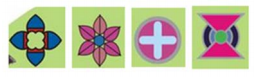# NCERT Solutions Class 5 Mathematics Chapter 5 Does it look the same

## Chapter 5 Does it look the same Class 5 NCERT Solutions

Class 5 Mathematics students should refer to the following NCERT questions with answers for Chapter 5 Does it look the same in standard 5. These NCERT Solutions with answers for Grade 5 Mathematics will come in exams and help you to score good marks

### Chapter 5 Does it look the same NCERT Solutions Class 5

1. Find out which letters in the English alphabet looks the same after a turn.

Ans. The letters in English alphabet which look the same after a turn are H, I, N, O, S, X and Z.

2. Which of these English words reads the same on half a turn? ZOOM, MOW, SWIMS, SIS, NOON

Ans. The English words MOW, SWIMS, SIS AND NOON read the same on half a turn.

3. Give half a turn to the numbers from 0 to 9. Find which of them stills look the same.

Ans. The numbers 0, 1 and 8 look the same after giving a turn to them.

4. Think of all 2, 3 and 4 digit numbers which look the same on half a turn.

Ans. All 2, 3 and 4 digit numbers which look the same on half a turn are: 2-digit numbers: 11, 88 3-digit numbers: 101, 111, 181, 808, 818, 888 4-digit numbers: 1001, 1111, 1881, 8008, 8118, 8888

5. Which among the following pictures will look the same on half a turn?

Ans. The following pictures will look the same on half a turn.## Books recommended by teachers

 NCERT Solutions Class 5 Mathematics Chapter 1 The Fish Tale
 NCERT Solutions Class 5 Mathematics Chapter 2 Shapes and Angles
 NCERT Solutions Class 5 Mathematics Chapter 3 How Many Squares
 NCERT Solutions Class 5 Mathematics Chapter 4 Parts and Wholes
 NCERT Solutions Class 5 Mathematics Chapter 5 Does it look the same
 NCERT Solutions Class 5 Mathematics Chapter 6 Be my multiply
 NCERT Solutions Class 5 Mathematics Chapter 7 Can you see the pattern
 NCERT Solutions Class 5 Mathematics Chapter 8 Mapping Your Way
 NCERT Solutions Class 5 Mathematics Chapter 9 Boxes And Sketches
 NCERT Solutions Class 5 Mathematics Chapter 10 Tenths And Hundredths
 NCERT Solutions Class 5 Mathematics Chapter 11 Area and its Boundary
 NCERT Solutions Class 5 Mathematics Chapter 12 Smart Charts
 NCERT Solutions Class 5 Mathematics Chapter 13 Ways of Multiply and Divide
 NCERT Solutions Class 5 Mathematics Chapter 14 How Big How Heavy